﻿ 复矩阵方程AXB = C的最小二乘Hermite解 Least Squares Hermitian Solution of Complex Matrix Equation AXB = C

Pure Mathematics
Vol.06 No.01(2016), Article ID:16846,8 pages
10.12677/PM.2016.61007

Least Squares Hermitian Solution of Complex Matrix Equation AXB = C

Peng Wang, Jianbo Chen

School of Mathematics and Computational Science, Wuyi University, Jiangmen Guangdong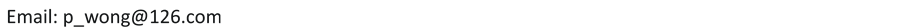Received: Dec. 23rd, 2015; accepted: Jan. 23rd, 2016; published: Jan. 28th, 2016ABSTRACT

Based on Moore-Penrose generalized inverse, by making use of matrix-vector production, an analytical expression of the least-squares Hermitian solution with the minimum-norm of complex matrix equation AXB = C is derived.

Keywords:Matrix Equation, Least-Square Solution, Moore-Penrose Inverse Generalized, Hermitian Solution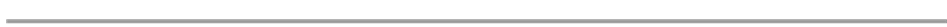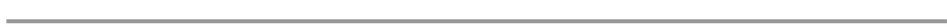1. 问题简述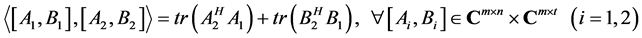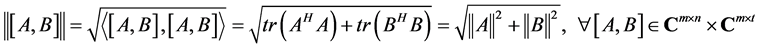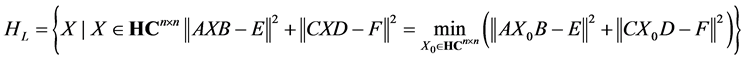(1.1)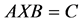(1.2)

2. Hilbert内积空间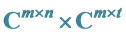中的一类最小二乘解问题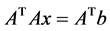(2.1)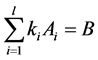(2.2)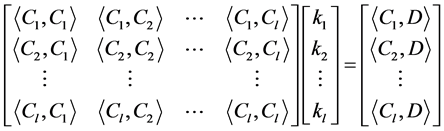(2.3)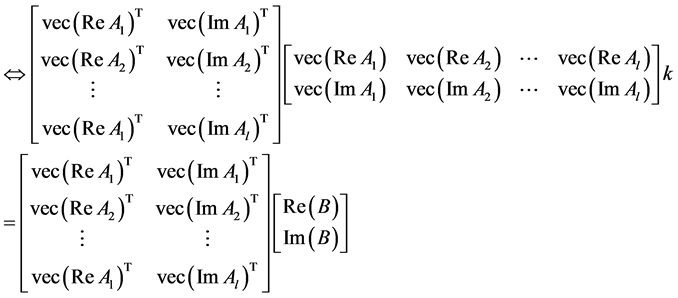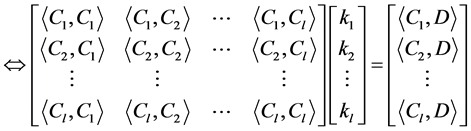.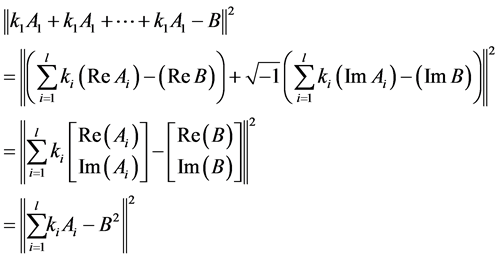3. 表达式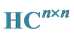空间上的结构形式

1)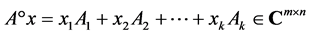2)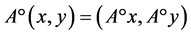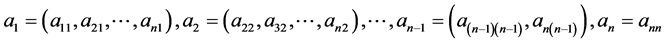，算子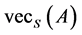定义为：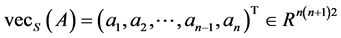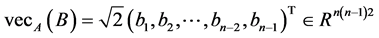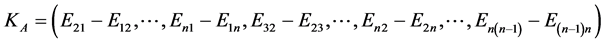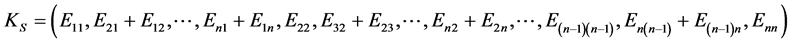1)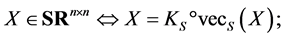2)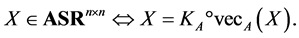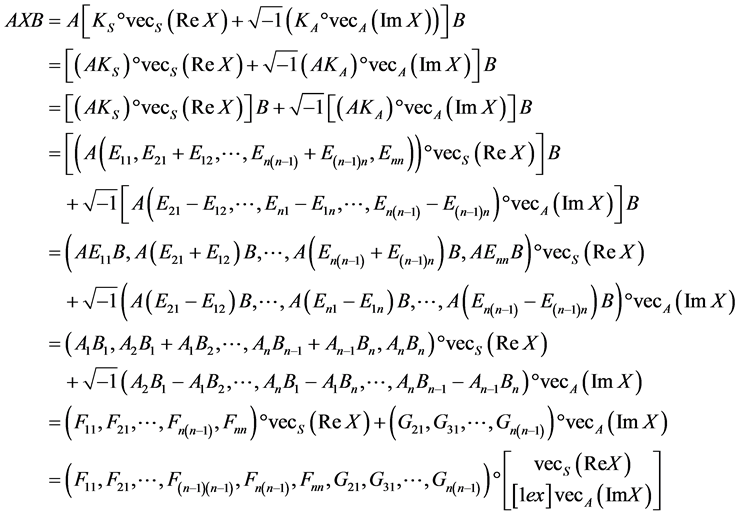4. 问题一的解的形式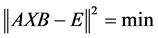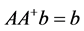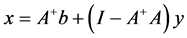，设：，其中，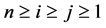。 (4.1)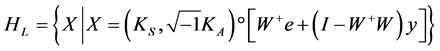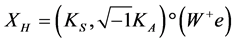。 (4.2)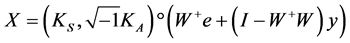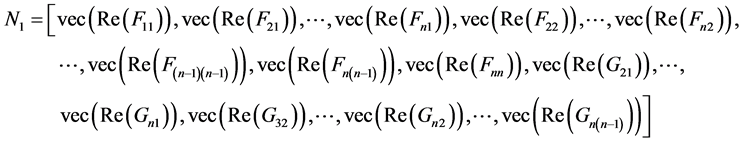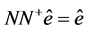(4.3)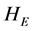是方程(1.2)的解集，则：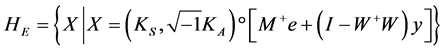5. 结论

Least Squares Hermitian Solution of Complex Matrix Equation AXB = C[J]. 理论数学, 2016, 06(01): 42-49. http://dx.doi.org/10.12677/PM.2016.61007

1. 1. Liao, A.-P. and Lei, Y. (2005) Least Squares Solution with the Mininum-Norm for the Matrix Equation (AXB, GXH) = (C, D). Computers & Mathematics with Applications, 50, 539-549. http://dx.doi.org/10.1016/j.camwa.2005.02.011

2. 2. Yuan, Y.-X. (2001) On the Two Classes of Best Approximation Problems. Mathematica Numerica Sinica, 23, 429- 436.

3. 3. Yuan, Y.-X. (2002) The Optimal Solution of Linear Matrix Equation by Matrix Decompositions. Mathematica Numerica Sinica, 24, 165-176.

4. 4. Yuan, S.-F., Liao, A.-P. and Lei, Y. (2008) Least Squares Hermitian Solution of the Matrix Equation (AXB, CXD) = (E, F) with the Least Norm over the Skew Field of Quaternions. Mathematical and Computer Modelling, 48, 91-100. http://dx.doi.org/10.1016/j.mcm.2007.08.009

5. 5. Ben-Israel, A. and Greville, T.N.E. (1974) Generalized Inverses: Theory and Applications. John Wiley and Sons, New York.

6. 6. Dehghan, M. and Hajarian, M. (2012) The generalized Sylvester Matrix Equations over the Generalized Bisymmetric and Skew-Symmetric Matrices. International Journal of Systems Science, 43, 1580-1590. http://dx.doi.org/10.1080/00207721.2010.549584

7. 7. Krishnaswamy, D. (2011) The Skew-Symmetric Ortho-Symmetric Solutions of the Matrix Equations A^* XA=D. International Journal of Algebra, 5, 1489-1504.

8. 8. Sheng, X.-P. and Chen, G.-L. (2010) An Iterative Method for the Symmetric and Skewsymmetric Solutions of a Linear Matrix Equation AXB + CYD = E. Journal of Computational and Applied Mathematics, 233, 3030-3040. http://dx.doi.org/10.1016/j.cam.2009.11.052

9. 9. Wang, Q.-W. and He, Z.-H. (2013) Solvability Conditions and General Solution for Mixed Sylvester Equations. Automatica, 49, 2713-2719. http://dx.doi.org/10.1016/j.automatica.2013.06.009

10. 10. Xiao, Q.F. (2012) The Hermitian R-Symmetric Solutions of the Matrix Equation AXA^*=B. International Journal of Algebra, 6, 903-911.

11. 11. Farid, F.O., Moslehian, M.S., Wang, Q.W. and Wu, Z.C. (2012) On the Hermitian Solutions to a System of Adjointable Operator Equations. Linear Algebra and Its Applications, 437, 1854-1891. http://dx.doi.org/10.1016/j.laa.2012.05.012

12. 12. Dong, C.Z., Wang, Q.W. and Zhang, Y.P. (2012) The Common Positive Solution to Adjointable Operators Equations with an Application. Journal of Mathematical Analysis and Applications, 396, 670-679. http://dx.doi.org/10.1016/j.jmaa.2012.07.001